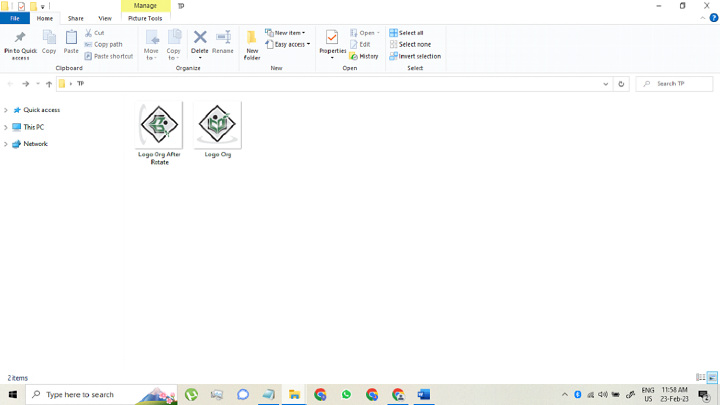# Java Program to Rotate an Image

A image file can rotated clockwise or anticlockwise direction. To rotate an image it is needed to download a random image file and save it in any folder on your system. Further, a .pdf file is required to create and rotate some degrees after opening the downloaded image in that particular .pdf file. For 90 degree rotations, anchor point of a new image help us to perform the rotation operation using the translate transformation in Java. The anchor point is the center for any particular image.

## Algorithm to Rotate an Image by Using Java

The "AffineTransformOp" class is the simplest way to rotate an image file by using Java. A user can load the image data as Buffered Image and apply the rotate operation using anchor point to produce the new BufferedImage for the next step. It is always recommended that to use JPEG files for these operation with JDeli(Filetype with wide range).

To rotate an image by using Java program a coder can use some in-built methods, like BufferedImage class and Color c.

Using this process, we need to load an image as BufferedImage into Java, then rotate the image using same function and save the data to a new file.

Now, let us discuss the algorithm to understand the above operation in a broad manner −

• Step 1 − Image read and write and import to the file class which presents the directory path.

• Step 2 − Use IOException: handle errors.

• Step 3 − For holding the particular image use the object called BufferedImage, a static method to save the data in RAM.

• Step 4 − Use ImageIO to run read and write operation.

• Step 5 − Use Graphics2D class, to render 2D shapes.

#### Syntax

Code declaration: public static Image rotate(Image image, double angle)


According to this syntax, there are some steps to understand −

• Parameters −

• Image − To perform the rotate operation

• Return − The rotated image file

Below processes can be used to rotate an image File by using Java Program −

• Step 1 − Load The Image File As BufferedImage In Java Environment To load an image file in Java −

BufferedImage image = ImageIO.read(new File("C:\path\to\image name.jpg"));

• Step 2 − Rotate the image by 90 degree

• To rotate an image file by 90 degree follow the below code −

final double rads = Math.toRadians(90);
final Rotate rotate = new Rotate(90);
BufferedImage rotatedImage = rotate.apply(image);

• Step 3  Save the image file

Use Java ImageIO
ImageIO.write(rotatedImage,"JPG",newFile("C:\path\to\rotatedImagename.jpg"));


### Example

import java.awt.*;
import java.awt.image.BufferedImage;
public class Main {
public static Image rotate(Image image, double angle) {
BufferedImage bufImg = toBufferedImage(image);
double sin = Math.abs(Math.sin(angle)), cos = Math.abs(Math.cos(angle));
int w = bufImg.getWidth(), h = bufImg.getHeight();
int neww = (int) Math.floor(w * cos + h * sin), newh = (int) Math.floor(h * cos + w * sin);
BufferedImage result = new BufferedImage(neww, newh, Transparency.TRANSLUCENT);
Graphics2D g = result.createGraphics();
g.translate((neww - w) / 2, (newh - h) / 2);
g.rotate(angle, w / 2, h / 2);
g.drawRenderedImage(bufImg, null);
g.dispose();
return result;
}
public static BufferedImage toBufferedImage(Image image) {
if (image instanceof BufferedImage) {
return (BufferedImage) image;
}
BufferedImage buff = new BufferedImage(image.getWidth(null), image.getHeight(null),
BufferedImage.TYPE_INT_ARGB);
Graphics2D g = buff.createGraphics();
g.drawImage(image, 0, 0, null);
g.dispose();
return buff;
}
}


### Rotation of an Image Using BufferedImage Function

By using a try-catch blocking method, we can handle the exceptions as they may disturb the flow of the code.

• Document Class − Document class is mainly used to present the pdf document. Here in this particular java program we need to create a document class by using ie.documentobj function. This function is used to open and close the pdf file.

• PDF Writer Class − The function supports the PDF, XML,RTF file generations to code the image file rotation. The directory function we use here is fileOutputStream() to handle a file for a java code.

• Output Function − output.pdf is a function class which denotes the output after performing the operation using the Java code. This function helps to get the output on the input provided.

• Functions −

• image class.imgage.scaleToFit() - The function helps us to set up a size in the input file preset.

• imageobj.setRotationDegrees() - the coder can use this to rotate the image in certain angle. It can be used as a parameter in the method we used.

• documentobj.open() - The function help the user to open a file at the time of operation.

• documentobj.close() - To close a .pdf file this function is used.

### Example

package JavaApplication29;
import java.io.FileOutputStream;
import com.itextpdf.text.Document;
import com.itextpdf.text.Image;
import com.itextpdf.text.PageSize;
import com.itextpdf.text.pdf.PdfWriter;

public class JavaApplication29 {
public static void main(String[] args) {
try {
Document documentobj = new Document(PageSize.A4, 20, 20, 20, 20);
PdfWriter.getInstance(documentobj, new FileOutputStream("output.pdf"));
documentobj.open();
Image imageobj = Image.getInstance("C:\Users\lenovo\Desktop\RDD\Logo Org.jpg");
imageobj.scaleToFit(200f, 200f);
imageobj.setRotationDegrees(90);
documentobj.close();
} catch (Exception e) {
System.out.println("Exception occurred");
}
}
}


### Output

By using the possible method is encoded in the program, the console shows the dimensions and execution pop-up. A new image is saved after rotation.## Conclusion

In this article, the above discussed processes help to rotate an image file. For 90 degree rotation, a program needs to set a new image, all parameters are needed to be changed. Since the anchor point remains in the center of the image and the operation is same both for clockwise and anti-clockwise rotation.

Updated on: 31-Mar-2023

1K+ Views